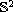Electron. J. Differential Equations, Vol. 2017 (2017), No. 189, pp. 1-40.

### Galerkin approximations of nonlinear optimal control problems in Hilbert spaces Mickael D. Chekroun, Axel Kroner, Honghu Liu

Abstract:
Nonlinear optimal control problems in Hilbert spaces are considered for which we derive approximation theorems for Galerkin approximations. Approximation theorems are available in the literature. The originality of our approach relies on the identification of a set of natural assumptions that allows us to deal with a broad class of nonlinear evolution equations and cost functionals for which we derive convergence of the value functions associated with the optimal control problem of the Galerkin approximations. This convergence result holds for a broad class of nonlinear control strategies as well. In particular, we show that the framework applies to the optimal control of semilinear heat equations posed on a general compact manifold without boundary. The framework is then shown to apply to geoengineering and mitigation of greenhouse gas emissions formulated here in terms of optimal control of energy balance climate models posed on the sphere.

Submitted April 2, 2017. Published July 28, 2017.
Math Subject Classifications: 35Q86, 35Q93, 35K58, 49J15, 49J20, 86-08.
Key Words: Nonlinear optimal control problems; Galerkin approximations; Greenhouse gas emissions; Energy balance models; Trotter-Kato approximations.

Show me the PDF file (522 KB), TEX file for this article.Mickaël D. Chekroun Department of Atmospheric & Oceanic Sciences University of California Los Angeles, CA 90095-1565, USA email: mchekroun@atmos.ucla.edu Axel Kröner Institut für Mathematik Humbolt-Universität Berlin 10099 Berlin, Germany email: axel.kroener@inria.fr Honghu Liu Department of Mathematics Virginia Polytechnic Institute and State University Blacksburg, Virginia 24061, USA email: hhliu@vt.edu